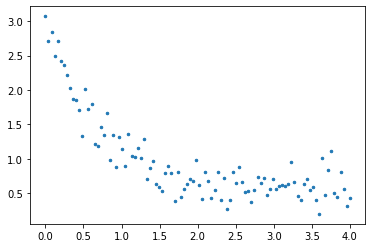Curve Fitting¶

In :
import numpy as np
import matplotlib.pyplot as plt
%matplotlib inline
from scipy.optimize    import fsolve
from scipy.interpolate import interp1d
from scipy.optimize    import curve_fit

Curve fitting is used to represent a set of data by a model function.

• The model function (the curve) can be a form meant for convenience. That is, we can represent the data using a function instead of the raw data itself.
• This can give some flexibility in data analysis (integration, differentiation, compact representation, etc.)
• Often, there is an underlying physical process assumed that created the data. Our model may be an equation representing this process.
• Find the best fit parameters for the curve.
• Either we want the parameters for their own sake,
• Or, we may just want parameters that allow the curve to fit well.

Recall the procedure in Excel

1. Use a trendline with a plot.
• Several model forms available: polynomial, linear, power law, logarithic, exponential, etc.
• Sometimes needed to massage the data to get in a model form.
2. Use the solver to minimize the sum square error by changing the model parameters.

Python

• Two methods:
1. polyfit for polynomial fits (including linear)
2. curve_fit for general curve fits

Polynomial fits¶

• p = np.polyfit(xg, yg, polynomial_order)
• np.polyval(p, xw)
• Set or obtain your given xg and yg data
• Get a polyfit object using polyfit
• Evaluate the polynomial at desired locations xw using polyval

Exercise¶

• Given the data below,
• Fit a third order polynomial.
• Print out the polynomial coefficients.
• Plot the data and the polynomial curve
• Use more points for the curve than just the xg data.
In :
xg = np.array([0., 1., 2., 3., 4., 5.])         # given x data
yg = np.array([0, 0.8, 0.9, 0.1, -0.8, -1.0])   # given y data

Excercise¶

• Given the data below for $2\le x\le 8$
• Fit a 6th order polynomial to the data.
• Plot the results for $0\le x\le 12$.
In :
xg = np.array([2, 3, 4, 5, 6, 7, 8])
yg = np.array([1, 2, 1, 2, 1, 2, 1])

Question¶

• What does this tell you about using the polynomial fit outside of the original range of the data?

General Curve Fits¶

We can fit a general function f(x; a, b, c) where f is a function of x with parameters a, b, c that we want to optimize for a set of given data.

Use curve_fit available from from scipy.optimize import curve_fit

• params, extras = curve_fit(f, xg, yg)

Example from scipy.org¶

Fit the following function to the given data $$f(x) = a\exp(-bx) + c$$

• Plot the data and the curve fit
In :
#-------- Set some given data (normally, this is already available)

xg = np.linspace(0,4,100)
yg = 2.5*np.exp(-1.3*xg)+0.5 + 0.2*np.random.normal(size=len(xg))

plt.plot(xg,yg,'.', markersize=5.0);Question¶

• What appears to be missing from this curve_fit function call?
• An initial guess for the parameters is not required, but it is possible to give this if desired.
• Use help(curve_fit) for details on this and other options.

Least Squares Approximation¶

• The goal of Least Squares Approximation is to fit a given function to a set of data.
• The function will have adjustable parameters, and we are finding the parameters that give the best fit, in some sense, to the data.
• The best fit can be defined in several ways, but the most popular is to form the sum square error between the function and the data points. The parameters are then modified to minimize the sum square error. ​
• Suppose
$$f(x;a,b,c) = ax^2 + bx + c.$$
• We have a set of points $(x_i,\,y_i)$, given, and a set of modeled points $(x_i,\,f(x_i;a,b,c))$. Denote $f(x_i;a,b,c)\equiv f_i$.
• The error for a given point is $\epsilon_i = f_i-y_i$.
• The total error is
$$E(a,b,c) = \sum_{i=0}^{N-1}\epsilon_i^2 = \sum_{i=0}^{N-1}(ax_i^2 + bx_i + c - y_i)^2.$$
• Now, we want to find the $a$, $b$, and $c$ that minimize $E$:
\begin{align*} \frac{\partial E}{\partial a} = &0 = \sum_i 2(ax_i^2 + bx_i + c - y_i)(x_i)^2, \\ \frac{\partial E}{\partial b} = &0 = \sum_i 2(ax_i^2 + bx_i + c - y_i)(x_i), \\ \frac{\partial E}{\partial c} = &0 = \sum_i 2(ax_i^2 + bx_i + c - y_i)(1) \end{align*}
• These give three linear equations that can be solved for $a$, $b$, and $c$.
• The equations are linear because the parameters appear linearly in the model equation.
• In general, the method works for arbitrary nonlinear model functions, but the resulting system of equations to solve may be nonlinear.

Example¶

• Consider the model function
$$f(x; a,b) = ax^b.$$$$E = \sum_i(ax_i^b - y_i)^2,$$\begin{align*} \frac{\partial E}{\partial a} = &0 = \sum_i2(ax_i^b-y_i)(x_i^b),\\ \frac{\partial E}{\partial b} = &0 = \sum_i2(ax_i^b-y_i)(ax_i^b\ln x_i). \end{align*}
• These last two equations are nonlinear.
• We can change variables by first linearizing the model function:
$$\ln f = \ln a + b\ln x.$$$$E = \sum_i(\underbrace{\ln a}_{\hat{a}} + b\ln x_i - \ln y_i)^2,$$$$E = \sum_i(\hat{a} + b\ln x_i - \ln y_i)^2.$$\begin{align*} \frac{\partial E}{\partial \hat{a}} = &0 = \sum_i2(\hat{a} + b\ln x_i - \ln y_i)(1) = 0, \\ \frac{\partial E}{\partial b} = &0 = \sum_i2(\hat{a} + b\ln x_i - \ln y_i)(\ln x_i) = 0. \\ \end{align*}
• These last two equations are linear in $\hat{a}$ and $b$.
• We can solve these and then recover $a=\exp(\hat{a})$.
In [ ]: